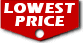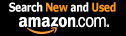# Probability Theory: A Concise Course (Dover Books on Mathematics) / Y.A. Rozanov

List Price: \$11.95
Our Price: \$9.66For Bulk orders
Quantity

Used Book Price: \$3.50
/ Y.A. Rozanov
Publisher: Dover Publications
Availability:Usually ships in 24 hours
Sales Rank: 74293
 ISBN-10: 0486635449ISBN-13: 9780486635446

Similar Books
 An Introduction to Information Theory: Symbols, Signals and Noise (Dover Books on Mathematics) Introduction to Graph Theory (Dover Books on Mathematics) Number Theory (Dover Books on Mathematics) Introduction to Topology: Third Edition (Dover Books on Mathematics) Linear Algebra (Dover Books on Mathematics) Principles of Statistics (Dover Books on Mathematics) Introductory Discrete Mathematics (Dover Books on Computer Science) Fourier Series (Dover Books on Mathematics) Ordinary Differential Equations (Dover Books on Mathematics) Theoretical Physics (Dover Books on Physics)

This book, a concise introduction to modern probability theory and certain of its ramifications, deals with a subject indispensable to natural scientists and mathematicians alike. Here the readers, with some knowledge of mathematics, will find an excellent treatment of the elements of probability together with numerous applications. Professor Y. A. Rozanov, an internationally known mathematician whose work in probability theory and stochastic processes has received wide acclaim, combines succinctness of style with a judicious selection of topics. His book is highly readable, fast-moving, and self-contained.
The author begins with basic concepts and moves on to combination of events, dependent events and random variables. He then covers Bernoulli trials and the De Moivre-Laplace theorem, which involve three important probability distributions (binomial, Poisson, and normal or Gaussian). The last three chapters are devoted to limit theorems, a detailed treatment of Markov chains, continuous Markov processes. Also included are appendixes on information theory, game theory, branching processes, and problems of optimal control. Each of the eight chapters and four appendixes has been equipped with numerous relevant problems (150 of them), many with hints and answers.
This volume is another in the popular series of fine translations from the Russian by Richard A. Silverman. Dr. Silverman, a former member of the Courant Institute of Mathematical Sciences of New York University and the Lincoln Laboratory of the Massachusetts Institute of Technology, is himself the author of numerous papers on applied probability theory. He has heavily revised the English edition and added new material. The clear exposition, the ample illustrations and problems, the cross-references, index, and bibliography make this book useful for self-study or the classroom.

Now you can buy Books online in USA,UK, India and more than 100 countries.
*Terms and Conditions apply
Disclaimer: All product data on this page belongs to.
No guarantees are made as to accuracy of prices and information.